## Example Questions

### Example Question #2 : Trapezoids

Trapezoid A and Trapezoid B are similar. The bases of Trapezoid A are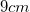and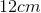. Trapezoid B has a smaller base of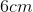. How long is the larger base of Trapezoid B?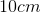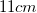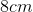Explanation:

Because the two trapezoids are similar, the ratio of their bases must be the same. Therefore, we must set up a cross-multiplication to solve for the missing base: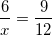, usingas the variable for the missing base.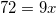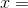Therefore, the length of the longer base of Trapezoid B is.